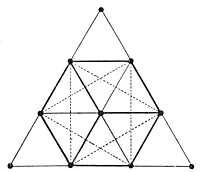Tetracyts is a sacred Pythagorean symbol, a mathematical model that was devised by the renowned mathematician, philosopher, sage and mystic, Pythagoras. It consists of 10 dots that are arranged in four rows (with four, three, two and one dot in each row) such that they make a triangular figure pointing upwards. ‘Tetracyts’ is a Greek word that means the number four.

In the figure, the first row has a single point that is representative of the Creator, the active principle, the divine power behind all creation and is associated with wisdom. The second row contains two points that represent the passive principle and are associated with friction, movement, impulse, strength, and courage. The third row with three points signifies the world coming out of the union of the above two, a union of physical and mental balance and is associated with harmony. The fourth row has four points that represent the four liberal arts & sciences that complete the world. These four points symbolize the four elements of earth, fire, air, and water.The arrangement depicted in the Tetracyts Symbol represents the organization of space, where the first row denotes zero dimension (a point), the second row denotes first dimension (a line joining two points), the third row denotes second dimension (a triangular plane), and the fourth row denotes the third dimension (a tetrahedron).

The Tetractys is a multi-layered, rich symbol that represents profoundly meaningful correspondences and relationships. According to the Pythagoreans, the archetypal powers of oneness, twoness, threeness & fourness could be used to understand and describe the sequence of creation and the nature of everything in the cosmos. Thus, the Tetracyts symbolizes the geometric, arithmetic and musical ratios that are the foundation on which the entire universe is built.

» Ajna

» Hung

» Halo

» Tau

» Chai

» Om

» Ankh

» Maat

» Dove

» Apex

» Yoni